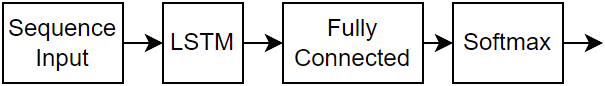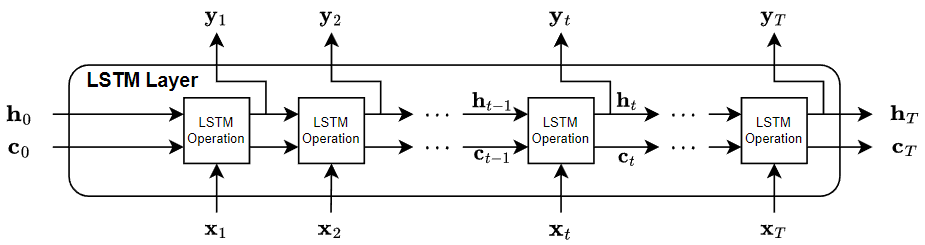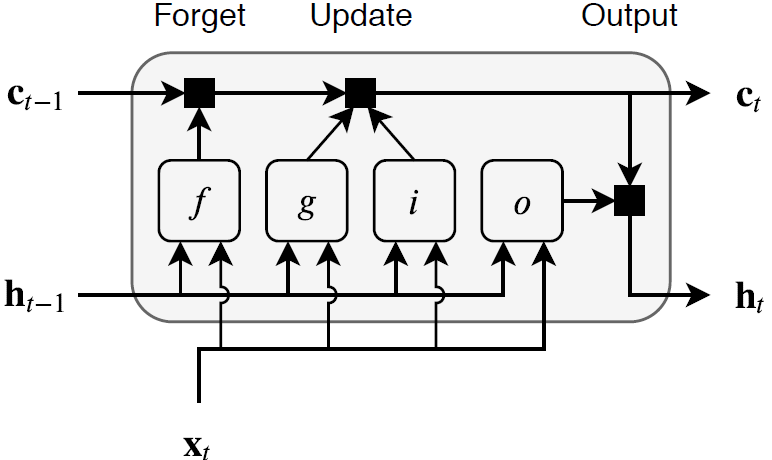## Long Short-Term Memory Networks

This topic explains how to work with sequence and time series data for classification and regression tasks using long short-term memory (LSTM) networks. For an example showing how to classify sequence data using an LSTM network, see Sequence Classification Using Deep Learning.

An LSTM network is a type of recurrent neural network (RNN) that can learn long-term dependencies between time steps of sequence data.

### LSTM Network Architecture

The core components of an LSTM network are a sequence input layer and an LSTM layer. A sequence input layer inputs sequence or time series data into the network. An LSTM layer learns long-term dependencies between time steps of sequence data.

This diagram illustrates the architecture of a simple LSTM network for classification. The network starts with a sequence input layer followed by an LSTM layer. To predict class labels, the network ends with a fully connected layer, a softmax layer, and a classification output layer.This diagram illustrates the architecture of a simple LSTM network for regression. The network starts with a sequence input layer followed by an LSTM layer. The network ends with a fully connected layer and a regression output layer.This diagram illustrates the architecture of a network for video classification. To input image sequences to the network, use a sequence input layer. To use convolutional layers to extract features, that is, to apply the convolutional operations to each frame of the videos independently, use a sequence folding layer followed by the convolutional layers, and then a sequence unfolding layer. To use the LSTM layers to learn from sequences of vectors, use a flatten layer followed by the LSTM and output layers.#### Classification LSTM Networks

To create an LSTM network for sequence-to-label classification, create a layer array containing a sequence input layer, an LSTM layer, a fully connected layer, a softmax layer, and a classification output layer.

Set the size of the sequence input layer to the number of features of the input data. Set the size of the fully connected layer to the number of classes. You do not need to specify the sequence length.

For the LSTM layer, specify the number of hidden units and the output mode `'last'`.

```numFeatures = 12; numHiddenUnits = 100; numClasses = 9; layers = [ ... sequenceInputLayer(numFeatures) lstmLayer(numHiddenUnits,'OutputMode','last') fullyConnectedLayer(numClasses) softmaxLayer classificationLayer];```

For an example showing how to train an LSTM network for sequence-to-label classification and classify new data, see Sequence Classification Using Deep Learning.

To create an LSTM network for sequence-to-sequence classification, use the same architecture as for sequence-to-label classification, but set the output mode of the LSTM layer to `'sequence'`.

```numFeatures = 12; numHiddenUnits = 100; numClasses = 9; layers = [ ... sequenceInputLayer(numFeatures) lstmLayer(numHiddenUnits,'OutputMode','sequence') fullyConnectedLayer(numClasses) softmaxLayer classificationLayer];```

#### Regression LSTM Networks

To create an LSTM network for sequence-to-one regression, create a layer array containing a sequence input layer, an LSTM layer, a fully connected layer, and a regression output layer.

Set the size of the sequence input layer to the number of features of the input data. Set the size of the fully connected layer to the number of responses. You do not need to specify the sequence length.

For the LSTM layer, specify the number of hidden units and the output mode `'last'`.

```numFeatures = 12; numHiddenUnits = 125; numResponses = 1; layers = [ ... sequenceInputLayer(numFeatures) lstmLayer(numHiddenUnits,'OutputMode','last') fullyConnectedLayer(numResponses) regressionLayer];```

To create an LSTM network for sequence-to-sequence regression, use the same architecture as for sequence-to-one regression, but set the output mode of the LSTM layer to `'sequence'`.

```numFeatures = 12; numHiddenUnits = 125; numResponses = 1; layers = [ ... sequenceInputLayer(numFeatures) lstmLayer(numHiddenUnits,'OutputMode','sequence') fullyConnectedLayer(numResponses) regressionLayer];```

For an example showing how to train an LSTM network for sequence-to-sequence regression and predict on new data, see Sequence-to-Sequence Regression Using Deep Learning.

#### Video Classification Network

To create a deep learning network for data containing sequences of images such as video data and medical images, specify image sequence input using the sequence input layer.

To use convolutional layers to extract features, that is, to apply the convolutional operations to each frame of the videos independently, use a sequence folding layer followed by the convolutional layers, and then a sequence unfolding layer. To use the LSTM layers to learn from sequences of vectors, use a flatten layer followed by the LSTM and output layers.

```inputSize = [28 28 1]; filterSize = 5; numFilters = 20; numHiddenUnits = 200; numClasses = 10; layers = [ ... sequenceInputLayer(inputSize,'Name','input') sequenceFoldingLayer('Name','fold') convolution2dLayer(filterSize,numFilters,'Name','conv') batchNormalizationLayer('Name','bn') reluLayer('Name','relu') sequenceUnfoldingLayer('Name','unfold') flattenLayer('Name','flatten') lstmLayer(numHiddenUnits,'OutputMode','last','Name','lstm') fullyConnectedLayer(numClasses, 'Name','fc') softmaxLayer('Name','softmax') classificationLayer('Name','classification')];```

Convert the layers to a layer graph and connect the `miniBatchSize` output of the sequence folding layer to the corresponding input of the sequence unfolding layer.

```lgraph = layerGraph(layers); lgraph = connectLayers(lgraph,'fold/miniBatchSize','unfold/miniBatchSize');```

For an example showing how to train a deep learning network for video classification, see Classify Videos Using Deep Learning.

#### Deeper LSTM Networks

You can make LSTM networks deeper by inserting extra LSTM layers with the output mode `'sequence'` before the LSTM layer. To prevent overfitting, you can insert dropout layers after the LSTM layers.

For sequence-to-label classification networks, the output mode of the last LSTM layer must be `'last'`.

```numFeatures = 12; numHiddenUnits1 = 125; numHiddenUnits2 = 100; numClasses = 9; layers = [ ... sequenceInputLayer(numFeatures) lstmLayer(numHiddenUnits1,'OutputMode','sequence') dropoutLayer(0.2) lstmLayer(numHiddenUnits2,'OutputMode','last') dropoutLayer(0.2) fullyConnectedLayer(numClasses) softmaxLayer classificationLayer];```

For sequence-to-sequence classification networks, the output mode of the last LSTM layer must be `'sequence'`.

```numFeatures = 12; numHiddenUnits1 = 125; numHiddenUnits2 = 100; numClasses = 9; layers = [ ... sequenceInputLayer(numFeatures) lstmLayer(numHiddenUnits1,'OutputMode','sequence') dropoutLayer(0.2) lstmLayer(numHiddenUnits2,'OutputMode','sequence') dropoutLayer(0.2) fullyConnectedLayer(numClasses) softmaxLayer classificationLayer];```

### Layers

LayerDescription`sequenceInputLayer`

A sequence input layer inputs sequence data to a network.`lstmLayer`

An LSTM layer learns long-term dependencies between time steps in time series and sequence data.`bilstmLayer`

A bidirectional LSTM (BiLSTM) layer learns bidirectional long-term dependencies between time steps of time series or sequence data. These dependencies can be useful when you want the network to learn from the complete time series at each time step.`gruLayer`

A GRU layer learns dependencies between time steps in time series and sequence data.`sequenceFoldingLayer`

A sequence folding layer converts a batch of image sequences to a batch of images. Use a sequence folding layer to perform convolution operations on time steps of image sequences independently.`sequenceUnfoldingLayer`

A sequence unfolding layer restores the sequence structure of the input data after sequence folding.`flattenLayer`

A flatten layer collapses the spatial dimensions of the input into the channel dimension.`wordEmbeddingLayer` (Text Analytics Toolbox)

A word embedding layer maps word indices to vectors.

### Classification, Prediction, and Forecasting

To classify or make predictions on new data, use `classify` and `predict`.

LSTM networks can remember the state of the network between predictions. The network state is useful when you do not have the complete time series in advance, or if you want to make multiple predictions on a long time series.

To predict and classify on parts of a time series and update the network state, use `predictAndUpdateState` and `classifyAndUpdateState`. To reset the network state between predictions, use `resetState`.

For an example showing how to forecast future time steps of a sequence, see Time Series Forecasting Using Deep Learning.

### Sequence Padding, Truncation, and Splitting

LSTM networks support input data with varying sequence lengths. When passing data through the network, the software pads, truncates, or splits sequences so that all the sequences in each mini-batch have the specified length. You can specify the sequence lengths and the value used to pad the sequences using the `SequenceLength` and `SequencePaddingValue` name-value pair arguments in `trainingOptions`.

After training the network, use the same mini-batch size and padding options when using the `classify`, `predict`, `classifyAndUpdateState`, `predictAndUpdateState`, and `activations` functions.

#### Sort Sequences by Length

To reduce the amount of padding or discarded data when padding or truncating sequences, try sorting your data by sequence length. To sort the data by sequence length, first get the number of columns of each sequence by applying `size(X,2)` to every sequence using `cellfun`. Then sort the sequence lengths using `sort`, and use the second output to reorder the original sequences.

```sequenceLengths = cellfun(@(X) size(X,2), XTrain); [sequenceLengthsSorted,idx] = sort(sequenceLengths); XTrain = XTrain(idx);```

The following figures show the sequence lengths of the sorted and unsorted data in bar charts.If you specify the sequence length `'longest'`, then the software pads the sequences so that all the sequences in a mini-batch have the same length as the longest sequence in the mini-batch. This option is the default.

The following figures illustrate the effect of setting `'SequenceLength'` to `'longest'`.#### Truncate Sequences

If you specify the sequence length `'shortest'`, then the software truncates the sequences so that all the sequences in a mini-batch have the same length as the shortest sequence in that mini-batch. The remaining data in the sequences is discarded.

The following figures illustrate the effect of setting `'SequenceLength'` to `'shortest'`.#### Split Sequences

If you set the sequence length to an integer value, then software pads all the sequences in a mini-batch to the nearest multiple of the specified length that is greater than the longest sequence length in the mini-batch. Then, the software splits each sequence into smaller sequences of the specified length. If splitting occurs, then the software creates extra mini-batches.

Use this option if the full sequences do not fit in memory. Alternatively, you can try reducing the number of sequences per mini-batch by setting the `'MiniBatchSize'` option in `trainingOptions` to a lower value.

If you specify the sequence length as a positive integer, then the software processes the smaller sequences in consecutive iterations. The network updates the network state between the split sequences.

The following figures illustrate the effect of setting `'SequenceLength'` to 5.The location of the padding and truncation can impact training, classification, and prediction accuracy. Try setting the `'SequencePaddingDirection'` option in `trainingOptions` to `'left'` or `'right'` and see which is best for your data.

Because LSTM layers process sequence data one time step at a time, when the layer `OutputMode` property is `'last'`, any padding in the final time steps can negatively influence the layer output. To pad or truncate sequence data on the left, set the `'SequencePaddingDirection'` option to `'left'`.

For sequence-to-sequence networks (when the `OutputMode` property is `'sequence'` for each LSTM layer), any padding in the first time steps can negatively influence the predictions for the earlier time steps. To pad or truncate sequence data on the right, set the `'SequencePaddingDirection'` option to `'right'`.

The following figures illustrate padding sequence data on the left and on the right.The following figures illustrate truncating sequence data on the left and on the right.### Normalize Sequence Data

To recenter training data automatically at training time using zero-center normalization, set the `Normalization` option of `sequenceInputLayer` to `'zerocenter'`. Alternatively, you can normalize sequence data by first calculating the per-feature mean and standard deviation of all the sequences. Then, for each training observation, subtract the mean value and divide by the standard deviation.

```mu = mean([XTrain{:}],2); sigma = std([XTrain{:}],0,2); XTrain = cellfun(@(X) (X-mu)./sigma,XTrain,'UniformOutput',false);```

### Out-of-Memory Data

Use datastores for sequence, time series, and signal data when data is too large to fit in memory or to perform specific operations when reading batches of data.

### Visualization

Investigate and visualize the features learned by LSTM networks from sequence and time series data by extracting the activations using the `activations` function. To learn more, see Visualize Activations of LSTM Network.

### LSTM Layer Architecture

This diagram illustrates the flow of a time series X with C features (channels) of length S through an LSTM layer. In the diagram, ${h}_{t}$ and ${c}_{t}$ denote the output (also known as the hidden state) and the cell state at time step t, respectively.The first LSTM block uses the initial state of the network and the first time step of the sequence to compute the first output and the updated cell state. At time step t, the block uses the current state of the network $\left({c}_{t-1},{h}_{t-1}\right)$ and the next time step of the sequence to compute the output and the updated cell state ${c}_{t}$.

The state of the layer consists of the hidden state (also known as the output state) and the cell state. The hidden state at time step t contains the output of the LSTM layer for this time step. The cell state contains information learned from the previous time steps. At each time step, the layer adds information to or removes information from the cell state. The layer controls these updates using gates.

The following components control the cell state and hidden state of the layer.

ComponentPurpose
Input gate (i)Control level of cell state update
Forget gate (f)Control level of cell state reset (forget)
Cell candidate (g)Add information to cell state
Output gate (o)Control level of cell state added to hidden state

This diagram illustrates the flow of data at time step t. The diagram highlights how the gates forget, update, and output the cell and hidden states.The learnable weights of an LSTM layer are the input weights W (`InputWeights`), the recurrent weights R (`RecurrentWeights`), and the bias b (`Bias`). The matrices W, R, and b are concatenations of the input weights, the recurrent weights, and the bias of each component, respectively. These matrices are concatenated as follows:

`$W=\left[\begin{array}{c}{W}_{i}\\ {W}_{f}\\ {W}_{g}\\ {W}_{o}\end{array}\right],R=\left[\begin{array}{c}{R}_{i}\\ {R}_{f}\\ {R}_{g}\\ {R}_{o}\end{array}\right],b=\left[\begin{array}{c}{b}_{i}\\ {b}_{f}\\ {b}_{g}\\ {b}_{o}\end{array}\right],$`

where i, f, g, and o denote the input gate, forget gate, cell candidate, and output gate, respectively.

The cell state at time step t is given by

`${c}_{t}={f}_{t}\odot {c}_{t-1}+{i}_{t}\odot {g}_{t},$`

where $\odot$ denotes the Hadamard product (element-wise multiplication of vectors).

The hidden state at time step t is given by

`${h}_{t}={o}_{t}\odot {\sigma }_{c}\left({c}_{t}\right),$`

where ${\sigma }_{c}$ denotes the state activation function. The `lstmLayer` function, by default, uses the hyperbolic tangent function (tanh) to compute the state activation function.

The following formulas describe the components at time step t.

ComponentFormula
Input gate${i}_{t}={\sigma }_{g}\left({W}_{i}{x}_{t}+\text{​}{\text{R}}_{i}{h}_{t-1}+{b}_{i}\right)$
Forget gate${f}_{t}={\sigma }_{g}\left({W}_{f}{x}_{t}+\text{​}{\text{R}}_{f}{h}_{t-1}+{b}_{f}\right)$
Cell candidate${g}_{t}={\sigma }_{c}\left({W}_{g}{x}_{t}+\text{​}{\text{R}}_{g}{h}_{t-1}+{b}_{g}\right)$
Output gate${o}_{t}={\sigma }_{g}\left({W}_{o}{x}_{t}+\text{​}{\text{R}}_{o}{h}_{t-1}+{b}_{o}\right)$

In these calculations, ${\sigma }_{g}$ denotes the gate activation function. The `lstmLayer` function, by default, uses the sigmoid function given by $\sigma \left(x\right)={\left(1+{e}^{-x}\right)}^{-1}$ to compute the gate activation function.

 Hochreiter, S., and J. Schmidhuber. "Long short-term memory." Neural computation. Vol. 9, Number 8, 1997, pp.1735–1780.